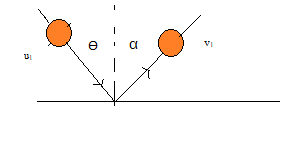# Coefficient of Restitution

## Coefficient of Restitution in one dimension

• Coefficient of Restitution is defined as the ratio of velocity of separation to the velocity of approach in the collisions
• Coefficient of Restitution is a unit less quantity and it is denoted by letter e. having values $0 \leq e \leq 1$
• If $u_1$,$u_2$ are the initial velocity and final velocities are $v_1$ and $v_2$, then Coefficient of Restitution can be defined as
$e = \frac {velocity \; of \; separation}{ velocity \; of \; approach}$
or
$e = \frac {v_2 - v_1}{u_1- u_2}$
• This is also known as Newton's Experimental Law of Impact
• The value of e is independent of the size and mass of the materials. It depends on the material only.
• The value of e is 0 for perfectly inelastic collision and 1 for elastic collision

## Coefficient of Restitution in two dimension or Oblique Collisions

• Line of impact is defined as the common normal to the surface of contact of the two colliding bodies
• In general Coefficient of Restitution is defined as the ratio of velocity of separation along the line of impact to the velocity of approach along the line of impact in the collisions
• So for Oblique Collisions, Newton 's Experimental Law of Impact or Coefficient of Restitution is applied for the components of velocity vector across the line of impact
• If a ball of initial velocity $u_1$ strikes a big ball at angle $\theta$ to the normal and it get rebounded at angle $\alpha$ with the normal with velocity $v_1$$e = \frac {velocity \; of \; separation}{ velocity \; of \; approach}$
or
$e = \frac {v_1 cos \alpha}{u_1 cos \theta}$
or $v_1 cos \alpha =e u_1 cos \theta$